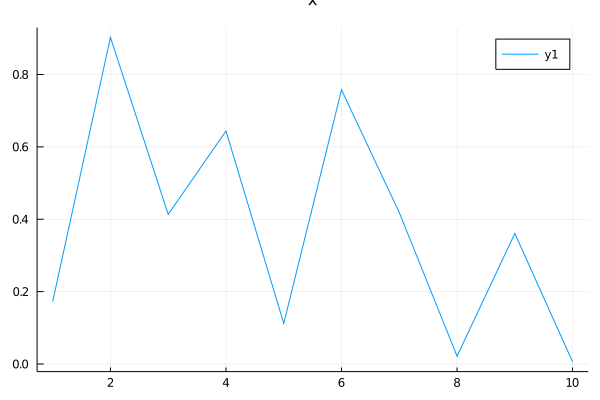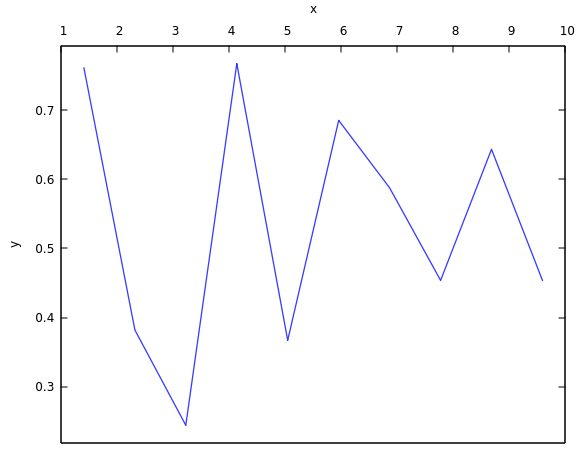# Putting xaxis ticks and labels on top (not bottom)

In a plot, the xticks and the xlabel go at the bottom. Can I directly put it on top rather than the bottom by somehow using `guide_position` somehow? or what does `guide_position` do?

1 Like

You can move the guide and the axis to the opposite position with the `mirror` attribute.

``````using Plots
plot(rand(10), xguide = "x", yguide = "y", xmirror = true)
```````guide_position` can be used to only move the guide, however this does not seem to work perfectly - it cuts the label in GR:

``````plot(rand(10), guide_position = :top, xguide = "x")
``````Both images above are with `gr()`. This does not work with `plotly()`. I am not sure about the other backends.

3 Likes
``````using Gaston
plot(rand(10), Axes(xtics=:off, x2tics = :on, x2label = :x, ylabel = :y))
``````2 Likes